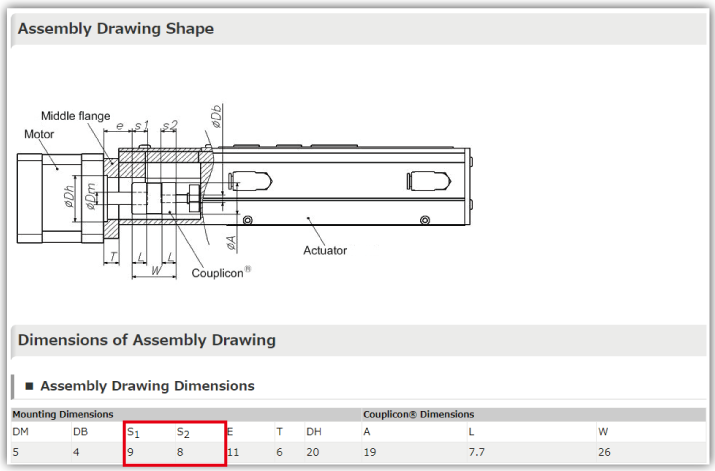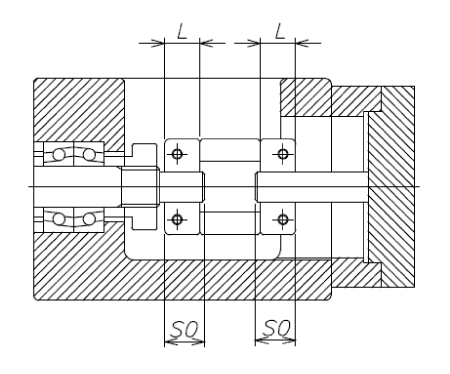#Date Published:Feb. 26. 2019

# Shaft Insertion Amount When Assembling the Coupling and Single-axis Actuator

## Calculation using Product Finder on Website

You can use the Product Finder on the NBK website to calculate the shaft insertion amount.

Select the actuator manufacturer/part number and the motor manufacturer/part number from the pulldown list on the right.From the pulldown results, select the coupling you want to use.S1 and S2 on the product details page are the shaft insertion amount dimensions.## Calculation by Hand

### 1. Calculate the distance between the two shafts

From the catalog, etc., for the actuator and motor you are using, calculate the respective lengths La and Lm of the shafts and the distance B between the two shafts when they are assembled.### 2. Calculate the temporary shaft insertion amount

Check the total coupling length W, and use conditional expression ① to calculate the temporary shaft insertion amount S0 on the motor side and actuator side.
S0=(W–B)/2 ･･･①

Check if the shaft lengths La and Lm on the actuator side and motor side respectively are at least 1 mm longer than the temporary shaft insertion amount S0.
(Conditional expressions ②③)
S0+1≦Lm ･･･②
S0+1≦La ･･･③### 3. Calculate the shaft insertion amount

Calculate the shaft insertion amounts Sa and Sm on the actuator side and motor side respectively.

##### A. If both conditional expressions ②③ are satisfied
Check if S0 is 70% or more of the coupling hub length L (conditional expression ④).
S0≧L×0.7 ･･･④
If conditional expression ④ is not satisfied, you will need to re-select the coupling.S0 becomes the shaft insertion amount Sa/Sm on the actuator and motor sides respectively.
Sa=S0
Sm=S0･･･⑤##### B. If neither of conditional expressions ②③ are satisfied
You will need to re-select the coupling.

##### C. If conditional expression ② is not satisfied(When the shaft length on the motor side Lm is shorter than the temporary shaft insertion amount S0+1 mm)
Check if the shaft length on the motor side Lm is at least 1 mm longer than 70% of the coupling hub length L (conditional expression ⑥).
Lm≧L×0.7+1 ･･･⑥
If conditional expression ⑥ is not satisfied, you will need to re-select the coupling.Use conditional expression ⑦ to calculate the insertion amount on the motor side Sm.
Sm=Lm–1 ･･･⑦

The shaft insertion amount on the actuator side Sa is defined by conditional expression ⑧.
Sa=W–B–Sm･･･⑧##### D. If conditional expression ③ is not satisfied (When the shaft length on the actuator side La is shorter than the temporary shaft insertion amount S0+1 mm)

Check if the shaft length on the actuator side La is at least 1 mm longer than 70% of the coupling hub length L (conditional expression ⑨).
La≧L×0.7+1 ･･･⑨
If conditional expression ⑨ is not satisfied, you will need to re-select the coupling.Use conditional expression ⑩ to calculate the shaft insertion amount on the actuator side Sa.
Sa=La–1 ･･･⑩

The insertion amount on the motor side Sm is defined by conditional expression ⑪.
Sm=W–B–Sa ･･･⑪### 4. Check the shaft insertion amount

Check if the shaft lengths La/Lm on the actuator side and motor side respectively are at least 1 mm longer than the shaft insertion amounts Sa/Sm on the actuator side and motor side respectively (conditional expressions ⑫⑬).
Sm+1≦Lm ･･･⑫
Sa+1≦La ･･･⑬

If conditional expressions ⑫⑬ are not satisfied, you will need to re-select the coupling.＊This procedure is just one example, and may vary depending on the actuator or motor type.